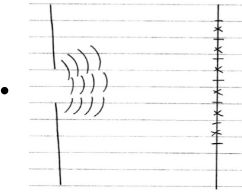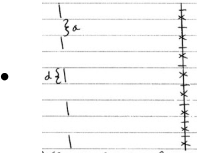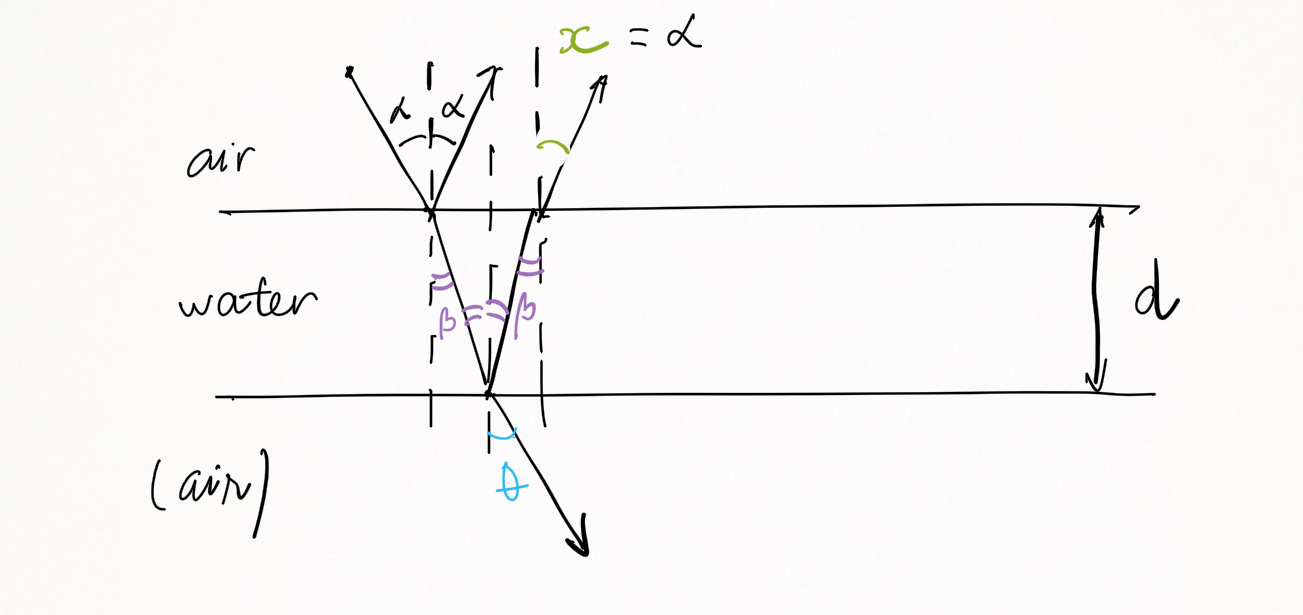MCAT Content / Light And Electromagnetic Radiation / Thin Films Diffraction Grating Single Slit Diffraction

### Thin films, diffraction grating, single-slit diffraction

Interference can also occur in single-slit diffraction (with a single slit), a diffraction grating (with multiple slits) and in thin films.

Single slit diffraction

Single slit diffraction is the phenomenon that occurs when waves pass through a narrow gap and bend, forming an interference pattern. This occurs when a coherent light source is forced through a small slit that creates a black-and-white pattern on the wall beyond the slit. A slit that is wider than a single wave will produce interference.Diffraction grating

A diffraction grating is an optical component with a periodic structure that splits and diffracts light into several beams travelling in different directions. The directions of these beams depend on the spacing of the grating and the wavelength of the light so that the grating acts as the dispersive element.

Diffraction grating: when there are many slits with aperture (a), separated by distance (d) between the slits.Thin Films

In thin films such as a layer of oil on the top of water, light waves can be reflected by the upper and lower boundaries of a thin film interfere with one another, either through constructive or destructive interference. This depends on the ratio between the thickness (d) of the film and the wavelength.Note that you can apply Snell’s Law to find the angle of Incidence (𝞪) is equal to the angle of Reflection (x): x = 𝞪. If the medium below water is air, the angle of refraction (θ) is also equal to the angle of Incidence (𝞪).Practice Questions

Imaging tissue structures using muon tomography

MCAT Official Prep (AAMC)

Practice Exam 4 C/P Section Question 46

Key Points

• Diffraction is interference that occurs in single slit or with multiple slits.

• Light behaves as a wave, with wavelength (λ) and a frequency (f). The wavelength (λ) must be much smaller than the aperture (a) for interference/diffraction to occur.

• The diffraction pattern depends on a, λ, f, d, and the medium between D.

•  Constructive or destructive interference can occur with thin films. This depends on the ratio between the thin film’s thickness d and the wavelength λ of light source.

Key Terms

Interference: (of waves) to be correlated with each other when overlapped or superposed.

Diffraction: the collision of light waves through 1 small slit

Coherent: of waves having the same direction, wavelength and phase, as light in a laser

Billing Information